# Construct a Complete N-ary Tree from given Postorder Traversal

• Difficulty Level : Hard
• Last Updated : 25 Jul, 2022

Given an array arr[] of size M that contains the post-order traversal of a complete N-ary tree, the task is to generate the N-ary tree and print its preorder traversal.

A complete tree is a tree where all the levels of the tree are completely filled, except may be for the last level but the nodes in the last level are as left as possible.

Examples:

Input: arr[] = {5, 6, 7, 2, 8, 9, 3, 4, 1}, N = 3
Output: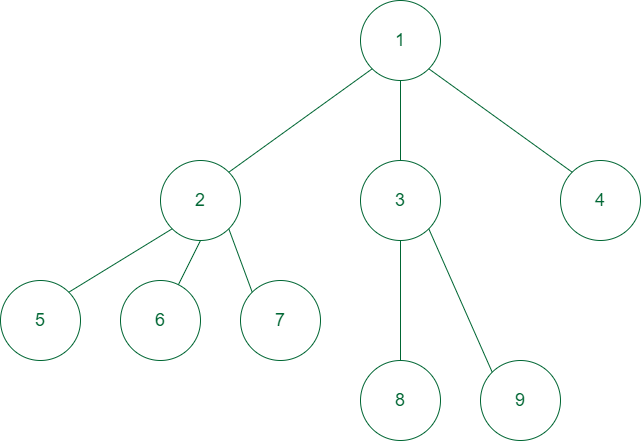The tree structure for above example

Input: arr[] = {7, 8, 9, 2, 3, 4, 5, 6, 1}, N = 5
Output: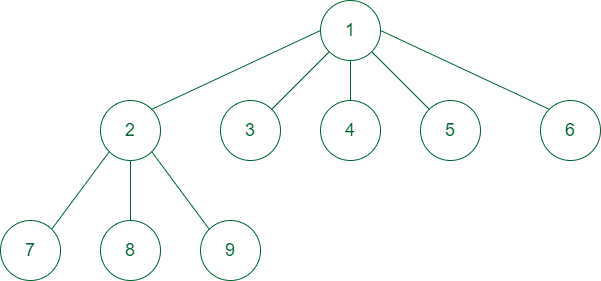Complete structure for the 2nd example

Approach: The approach to the problem is based on the following facts about the complete N-ary tree:

Say any subtree of the complete N-ary tree has a height H. So there are total H+1 levels numbered from 0 to H.

Total number of nodes in first H levels are N0 + N1 + N2 + . . . + NH-1 = (NH – 1)/(N – 1)

The maximum possible nodes in the last level is NH
So if the last level of the subtree has at least NH nodes, then total nodes in the last level of the subtree is  (NH – 1)/(N – 1) +  NH

The height H can be calculated as ceil[logN( M*(N-1) +1)] – 1 because
N-ary complete binary tree there can have at max (NH+1 – 1)/(N – 1)] nodes

Since the given array contains the post-order traversal, the last element of the array will always be the root node. Now based on the above observation the remaining array can be partitioned into a number of subtrees of that node.

Follow the steps mentioned below to solve the problem:

1. The last element of the array is the root of the tree.
2. Now break the remaining array into subarrays which represent the total number of nodes in each subtree.
3. Each of these subtrees surely has a height of H-2 and based on the above observation if any subtree has more than (NH-1 – 1)/(N – 1) nodes then it has a height of H-1.
4. To calculate the number of nodes in subtrees follow the below cases:
• If the last level has more than NH-1 nodes, then all levels in this subtree are full and the subtree has (NH-1-1)/(N-1) + NH-1 nodes.
• Otherwise, the subtree will have (NH-1-1)/(N-1) + L nodes where L is the number of nodes in the last level.
• L can be calculated as L = M – (NH – 1)/(N – 1)
5. To generate each subarray repeatedly apply the 2nd to 4th steps adjusting the size (M) and height (H) accordingly for each subtree.
6. Return the preorder traversal of the tree formed in this way

Follow the illustration below for a better understanding

Illustration:

Consider the example: arr[] = {5, 6, 7, 2, 8, 9, 3, 4, 1}, N = 3.

So height (H) = ceil[ log3(9*2 + 1) ] – 1 = ceil[log319] – 1 = 3 – 1 = 2 and L = 9 – (32 – 1)/2 = 5.

1st step: So the root = 11st step of forming the tree

2nd step: The remaining array will be broken into subtrees. For the first subtree H = 2.
5 > 32-1 i.e., L > 3. So the last level is completely filled and the number of nodes in the first subtree = (3-1)/2 + 3 = 4 [calculated using the formulae shown above].

The first subtree contains element = {5, 6, 7, 2}. The remaining part is {8, 9, 3, 4}
The root of the subtree = 2.

Now when the subtree for 5, 6, and 7 are calculated using the same method they don’t have any children and are the leaf nodes themselves.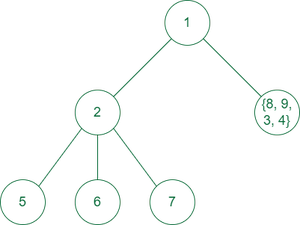2nd step of generating the tree

3rd step: Now L is updated to 2.
2 < 3. So using the above formula, number of nodes in the second subtree is 1 + 2 = 3.

So the second subtree have elements {8, 9, 3} and the remaining part is {4} and 3 is the root of the second subtree.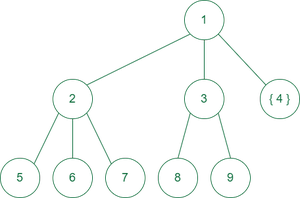3nd step of generating the tree

4th step: L = 0 now and the only element of the subtree is {4} itself.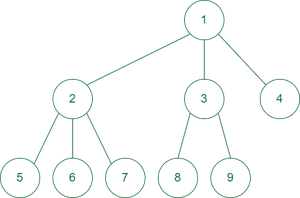Final structure of tree

After this step the tree is completely built.

Below is the implementation of the above approach:

## C++

 `// C++ code to implement the approach` `#include ``using` `namespace` `std;` `// Node Class``template` `<``typename` `T> ``class` `Node {``public``:``    ``Node(T data);` `    ``// Get the first child of the Node``    ``Node* get_first_child() ``const``;` `    ``// Get the Next Sibling of The node``    ``Node* get_next_sibling() ``const``;` `    ``// Sets the next sibling of the node``    ``void` `set_next_sibling(Node* node);` `    ``// Sets the next child of the node``    ``void` `set_next_child(Node* node);` `    ``// Returns the data the node contains``    ``T get_data();` `private``:``    ``T data;` `    ``// We use the first child/next sibling representation``    ``// to represent the Tree``    ``Node* first_child;``    ``Node* next_sibling;``};` `// Using template for generic usage``template` `<``typename` `T> Node::Node(T data)``{``    ``first_child = NULL;``    ``next_sibling = NULL;``    ``this``->data = data;``}` `// Function to get the first child``template` `<``typename` `T>``Node* Node::get_first_child() ``const``{``    ``return` `first_child;``}` `// Function to get the siblings``template` `<``typename` `T>``Node* Node::get_next_sibling() ``const``{``    ``return` `next_sibling;``}` `// Function to set next sibling``template` `<``typename` `T>``void` `Node::set_next_sibling(Node* node)``{``    ``if` `(next_sibling == NULL) {``        ``next_sibling = node;``    ``}``    ``else` `{``        ``next_sibling->set_next_sibling(node);``    ``}``}` `// Function to get the data``template` `<``typename` `T> T Node::get_data() { ``return` `data; }` `// Function to set the child node value``template` `<``typename` `T>``void` `Node::set_next_child(Node* node)``{``    ``if` `(first_child == NULL) {``        ``first_child = node;``    ``}``    ``else` `{``        ``first_child->set_next_sibling(node);``    ``}``}` `// Function to construct the tree``template` `<``typename` `T>``Node* Construct(T* post_order_arr, ``int` `size, ``int` `k)``{``    ``Node* Root = ``new` `Node(post_order_arr[size - 1]);``    ``if` `(size == 1) {``        ``return` `Root;``    ``}``    ``int` `height_of_tree``        ``= ``ceil``(log2(size * (k - 1) + 1) / log2(k)) - 1;``    ``int` `nodes_in_last_level``        ``= size - ((``pow``(k, height_of_tree) - 1) / (k - 1));``    ``int` `tracker = 0;``    ``while` `(tracker != (size - 1)) {``        ``int` `last_level_nodes``            ``= (``pow``(k, height_of_tree - 1)``               ``> nodes_in_last_level)``                  ``? nodes_in_last_level``                  ``: (``pow``(k, height_of_tree - 1));``        ``int` `nodes_in_next_subtree``            ``= ((``pow``(k, height_of_tree - 1) - 1) / (k - 1))``              ``+ last_level_nodes;` `        ``Root->set_next_child(``            ``Construct(post_order_arr + tracker,``                      ``nodes_in_next_subtree, k));` `        ``tracker = tracker + nodes_in_next_subtree;``        ``nodes_in_last_level``            ``= nodes_in_last_level - last_level_nodes;``    ``}``    ``return` `Root;``}` `// Function to print the preorder traversal``template` `<``typename` `T> ``void` `preorder(Node* Root)``{``    ``if` `(Root == NULL) {``        ``return``;``    ``}``    ``cout << Root->get_data() << ``"  "``;``    ``preorder(Root->get_first_child());``    ``preorder(Root->get_next_sibling());``}` `// Driver code``int` `main()``{``    ``int` `M = 9, N = 3;``    ``int` `arr[] = { 5, 6, 7, 2, 8, 9, 3, 4, 1 };``  ` `    ``// Function call``    ``preorder(Construct(arr, M, N));``    ``return` `0;``}`

## Java

 `// Java code to implement the approach` `import` `java.io.*;``import` `java.util.*;` `class` `GFG``{` `  ``// Function to calculate the``  ``// log base 2 of an integer``  ``public` `static` `int` `log2(``int` `N)``  ``{` `    ``// calculate log2 N indirectly``    ``// using log() method``    ``int` `result = (``int``)(Math.log(N) / Math.log(``2``));` `    ``return` `result;``  ``}` `  ``// Node Class``  ``public` `static` `class` `Node {``    ``int` `data;` `    ``// We use the first child/next sibling``    ``// representation to represent the Tree``    ``Node first_child;``    ``Node next_sibling;` `    ``// Initiallizing Node using Constructor``    ``public` `Node(``int` `data)``    ``{``      ``this``.first_child = ``null``;``      ``this``.next_sibling = ``null``;``      ``this``.data = data;``    ``}` `    ``// Function to get the first child``    ``public` `Node get_first_child()``    ``{``      ``return` `this``.first_child;``    ``}` `    ``// Function to get the siblings``    ``public` `Node get_next_sibling()``    ``{``      ``return` `this``.next_sibling;``    ``}` `    ``// Function to set the next sibling``    ``public` `void` `set_next_sibling(Node node)``    ``{``      ``if` `(``this``.next_sibling == ``null``) {``        ``this``.next_sibling = node;``      ``}``      ``else` `{``        ``this``.next_sibling.set_next_sibling(node);``      ``}``    ``}` `    ``// Function to get the data``    ``public` `int` `get_data() { ``return` `this``.data; }` `    ``// Function to set the child node values``    ``public` `void` `set_next_child(Node node)``    ``{``      ``if` `(``this``.first_child == ``null``) {``        ``this``.first_child = node;``      ``}``      ``else` `{``        ``this``.first_child.set_next_sibling(node);``      ``}``    ``}``  ``}` `  ``public` `static` `void` `main(String[] args)``  ``{``    ``int` `M = ``9``, N = ``3``;``    ``int``[] arr = { ``5``, ``6``, ``7``, ``2``, ``8``, ``9``, ``3``, ``4``, ``1` `};` `    ``// Function call``    ``preorder(Construct(arr, ``0``, M, N));``  ``}` `  ``// Function to print the preorder traversal``  ``public` `static` `void` `preorder(Node Root)``  ``{``    ``if` `(Root == ``null``) {``      ``return``;``    ``}``    ``System.out.println(Root.get_data() + ``"  "``);``    ``preorder(Root.get_first_child());``    ``preorder(Root.get_next_sibling());``  ``}` `  ``// Function to construct the tree``  ``public` `static` `Node Construct(``int``[] post_order_arr,``                               ``int` `tracker, ``int` `size,``                               ``int` `k)``  ``{``    ``Node Root = ``new` `Node(post_order_arr[tracker+size - ``1``]);``    ``if` `(size == ``1``) {``      ``return` `Root;``    ``}``    ``int` `height_of_tree``      ``= (``int``)Math.ceil(log2(size * (k - ``1``) + ``1``)``                       ``/ log2(k))``      ``- ``1``;``    ``int` `nodes_in_last_level``      ``= size``      ``- (((``int``)Math.pow(k, height_of_tree) - ``1``)``         ``/ (k - ``1``));``    ``int` `x=tracker;``    ``while` `(tracker != (size - ``1``)) {``      ``int` `last_level_nodes``        ``= ((``int``)Math.pow(k, height_of_tree - ``1``)``           ``> nodes_in_last_level)``        ``? nodes_in_last_level``        ``: ((``int``)Math.pow(k,``                         ``height_of_tree - ``1``));``      ``int` `nodes_in_next_subtree``        ``= (((``int``)Math.pow(k, height_of_tree - ``1``)``            ``- ``1``)``           ``/ (k - ``1``))``        ``+ last_level_nodes;` `      ``Root.set_next_child(Construct(``        ``post_order_arr, tracker, nodes_in_next_subtree, k));` `      ``tracker = tracker + nodes_in_next_subtree;``      ``nodes_in_last_level``        ``= nodes_in_last_level - last_level_nodes;``    ``}``    ``return` `Root;``  ``}``}`

## Python3

 `# Python code to implement the approach``import` `math` `# Node Class``class` `Node:``    ``# We use the first child/next sibling representation to represent the Tree``    ``def` `__init__(``self``, data):``        ``self``.data ``=` `data``        ``self``.first_child ``=` `None``        ``self``.next_sibling ``=` `None` `    ``# Function to get the first child``    ``def` `get_first_child(``self``):``        ``return` `self``.first_child` `    ``# Function to get the siblings``    ``def` `get_next_sibling(``self``):``        ``return` `self``.next_sibling` `    ``# Function to set next sibling``    ``def` `set_next_sibling(``self``, node):``        ``if` `self``.next_sibling ``is` `None``:``            ``self``.next_sibling ``=` `node``        ``else``:``            ``self``.next_sibling.set_next_sibling(node)` `    ``# Function to set the child node value``    ``def` `set_next_child(``self``, node):``        ``if` `self``.first_child ``is` `None``:``            ``self``.first_child ``=` `node``        ``else``:``            ``self``.first_child.set_next_sibling(node)` `    ``# Function to get the data``    ``def` `get_data(``self``):``        ``return` `self``.data` `# Function to construct the tree``def` `Construct(post_order_arr, size, k):` `    ``Root ``=` `Node(post_order_arr[size ``-` `1``])``    ``if` `size ``=``=` `1``:``        ``return` `Root``    ``height_of_tree ``=` `math.ceil(``        ``math.log(size ``*` `(k ``-` `1``) ``+` `1``, ``2``) ``/` `math.log(k, ``2``)) ``-` `1` `    ``nodes_in_last_level ``=` `size ``-` `((``pow``(k, height_of_tree) ``-` `1``) ``/` `(k ``-` `1``))``    ``tracker ``=` `0` `    ``while` `tracker !``=` `(size ``-` `1``):``        ``if` `pow``(k, height_of_tree``-``1``) > nodes_in_last_level:``            ``last_level_nodes ``=` `int``(nodes_in_last_level)``        ``else``:``            ``last_level_nodes ``=` `int``((``pow``(k, height_of_tree ``-` `1``)))` `        ``nodes_in_next_subtree ``=` `int``(``            ``((``pow``(k, height_of_tree ``-` `1``) ``-` `1``) ``/` `(k ``-` `1``)) ``+` `last_level_nodes)` `        ``Root.set_next_child(``            ``Construct(post_order_arr[tracker:], nodes_in_next_subtree, k))` `        ``tracker ``=` `tracker ``+` `nodes_in_next_subtree``        ``nodes_in_last_level ``=` `nodes_in_last_level ``-` `last_level_nodes` `    ``return` `Root` `# Function to print the preorder traversal``def` `preorder(root):``    ``if` `root ``=``=` `None``:``        ``return``    ``print``(root.get_data(), end``=``" "``)``    ``preorder(root.get_first_child())``    ``preorder(root.get_next_sibling())`  `# Driver code``if` `__name__ ``=``=` `'__main__'``:``    ``M ``=` `9``    ``N ``=` `3``    ``arr ``=` `[``5``, ``6``, ``7``, ``2``, ``8``, ``9``, ``3``, ``4``, ``1``]` `    ``# Function call``    ``preorder(Construct(arr, M, N))` `# This code is contributed by Tapesh(tapeshdua420)`

## C#

 `// C# code to implement the approach``using` `System;``using` `System.Collections.Generic;``using` `System.Linq;` `// Node Class``class` `Program {` `    ``public` `class` `Node {``        ``int` `data;` `        ``// We use the first child/next sibling``        ``// representation to represent the Tree``        ``Node first_child;``        ``Node next_sibling;` `        ``public` `Node(``int` `data)``        ``{``            ``this``.first_child = ``null``;``            ``this``.next_sibling = ``null``;``            ``this``.data = data;``        ``}` `        ``// Function to get the first child``        ``public` `Node get_first_child()``        ``{``            ``return` `this``.first_child;``        ``}` `        ``// Function to get the siblings``        ``public` `Node get_next_sibling()``        ``{``            ``return` `this``.next_sibling;``        ``}` `        ``// Function to set next sibling``        ``public` `void` `set_next_sibling(Node node)``        ``{``            ``if` `(``this``.next_sibling == ``null``) {``                ``this``.next_sibling = node;``            ``}``            ``else` `{``                ``this``.next_sibling.set_next_sibling(node);``            ``}``        ``}` `        ``// Function to get the data``        ``public` `int` `get_data() { ``return` `this``.data; }` `        ``// Function to set the child node value``        ``public` `void` `set_next_child(Node node)``        ``{``            ``if` `(``this``.first_child == ``null``) {``                ``this``.first_child = node;``            ``}``            ``else` `{``                ``this``.first_child.set_next_sibling(node);``            ``}``        ``}``    ``}``    ``// Driver code``    ``public` `static` `void` `Main(``string``[] args)``    ``{``        ``int` `M = 9, N = 3;``        ``int``[] arr = { 5, 6, 7, 2, 8, 9, 3, 4, 1 };` `        ``preorder(Construct(arr, M, N));``    ``}``    ``// Function to print the preorder traversal``    ``public` `static` `void` `preorder(Node Root)``    ``{``        ``if` `(Root == ``null``) {``            ``return``;``        ``}``        ``Console.Write(Root.get_data() + ``"  "``);``        ``preorder(Root.get_first_child());``        ``preorder(Root.get_next_sibling());``    ``}` `    ``// Function to construct the tree``    ``public` `static` `Node Construct(``int``[] post_order_arr,``                                 ``int` `size, ``int` `k)``    ``{``        ``Node Root = ``new` `Node(post_order_arr[size - 1]);``        ``if` `(size == 1) {``            ``return` `Root;``        ``}` `        ``int` `height_of_tree = (``int``)Math.Ceiling(``            ``(``double``)(Math.Log(size * (k - 1) + 1, 2)``                     ``/ Math.Log(k, 2))``            ``- 1);``        ``// Console.WriteLine(height_of_tree);` `        ``int` `nodes_in_last_level``            ``= size``              ``- (((``int``)Math.Pow(k, height_of_tree) - 1)``                 ``/ (k - 1));``        ``int` `tracker = 0;``        ``while` `(tracker != (size - 1)) {``            ``int` `last_level_nodes``                ``= ((``int``)Math.Pow(k, height_of_tree - 1)``                   ``> nodes_in_last_level)``                      ``? nodes_in_last_level``                      ``: ((``int``)Math.Pow(k,``                                       ``height_of_tree - 1));` `            ``int` `nodes_in_next_subtree``                ``= (``int``)((Math.Pow(k, height_of_tree - 1)``                         ``- 1)``                        ``/ (k - 1))``                  ``+ last_level_nodes;` `            ``Root.set_next_child(``                ``Construct((post_order_arr.Skip(tracker))``                              ``.Cast<``int``>()``                              ``.ToArray(),``                          ``nodes_in_next_subtree, k));` `            ``tracker = tracker + nodes_in_next_subtree;``            ``nodes_in_last_level``                ``= nodes_in_last_level - last_level_nodes;``        ``}``        ``return` `Root;``    ``}``}` `// This Code is contributed by Tapesh(tapeshdua420)`

Output

`1  2  5  6  7  3  8  9  4  `

Time Complexity: O(M)
Auxiliary Space: O(M) for building the tree

My Personal Notes arrow_drop_up